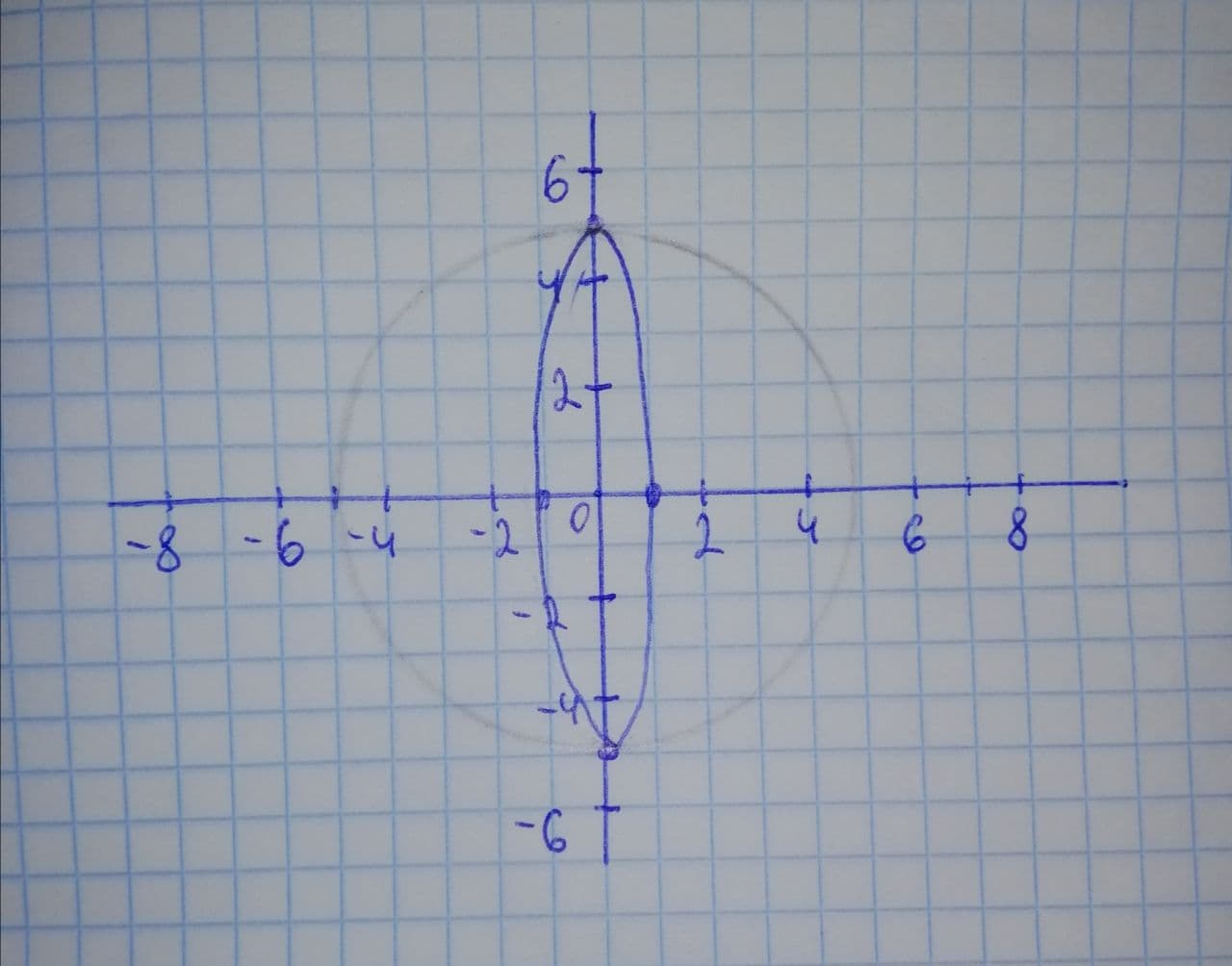Find the solution set for the system x^{2}+y^{2}=25 25x^{2}+y^{2}=25 by graphing bothtbbfiladelfia6l 2021-11-22 Answered
Find the solution set for the system
$$\displaystyle{x}^{{{2}}}+{y}^{{{2}}}={25}$$
$$\displaystyle{25}{x}^{{{2}}}+{y}^{{{2}}}={25}$$
by graphing both of the system’s equations in the same rectangular coordinate system and finding points of intersection. Check all solutions in both equations.

• Questions are typically answered in as fast as 30 minutes

Solve your problem for the price of one coffee

• Math expert for every subject
• Pay only if we can solve itTheirl1972
Step 1
we have to write the solution set for the system of
$$\displaystyle{x}^{{{2}}}+{y}^{{{2}}}={25}$$
$$\displaystyle{25}{x}^{{{2}}}+{y}^{{{2}}}={25}$$
By drawing the graph. We will first draw the graph and then through the graphical analysis we will draw the conclusion for the solution set.
Step 2
$$\displaystyle{x}^{{{2}}}+{y}^{{{2}}}={25}$$
$$\displaystyle{25}{x}^{{{2}}}+{y}^{{{2}}}={25}$$the solution are $$\displaystyle{S}={\left\lbrace{\left({0},\ {5}\right)},\ {\left({0},\ −{5}\right)}\right\rbrace}$$